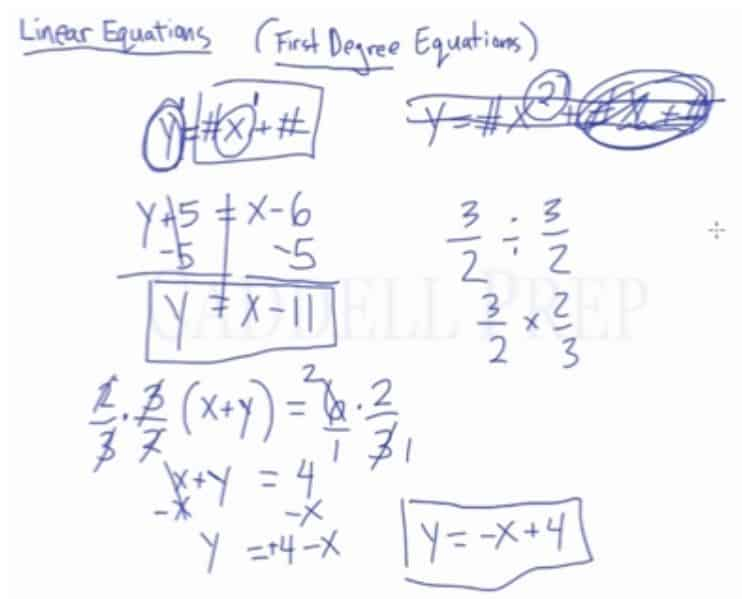In this video, we will be learning how to solve for one variable in terms of another in linear equations, such as solving for y in terms of x. After you finish this lesson, view all of our Algebra 1 lessons and practice problems.

Example of Solving a Linear Equation$\frac{3}{2}(x+y)=6$$\frac{3}{2}(x+y)\cdot\frac{2}{3}=6\cdot\frac{2}{3}\leftarrow$ Multiply both sides by$\frac{2}{3}$ to isolate x and y$(x+y)-x=4-x\leftarrow$ Subtract x from both sides to isolate y$y=-x+4\leftarrow$ Write in$y=mx+b$ formExample 1$\dfrac{y}{2}-8x=6$

First, we must get rid of$\dfrac{1}{2}$. So, we multiply it by its reciprocal.$2(\dfrac{y}{2}-8x)=(6)(2)$$y-16x=12$

Let’s add$16x$ to both sides to keep the format$y=ax+b$$y-16x+16x=12+16x$

Therefore,$y=16x+12$

Example 2$3(6x+3y)=9$

First, distribute$3$ to each of the terms inside the parenthesis$3(6x)+3(3y)=9$$18x+9y=9$

Then, subtract$18x$ to both sides$18x+9y-18x=9-16x$$9y=-18x+9$

We have to get rid of$9$ in$9y$ to keep the format$y=ax+b$

So, we multiply it by its reciprocal$\dfrac{1}{9}(9y)=(-18x+9)\dfrac{1}{9}$

Therefore,$y=-2x+1$

Video-Lesson Transcript

In this lesson, we’ll go over linear equations also known as first degree equations.

The basic format of a linear equation is$y = ax + b$, where$a$ and$b$ are numbers.

The first thing is that we have two variables$y$ and$x$.

A very important reminder about linear equation is why it’s called first-degree equation.

It’s because the exponent for the variables –$y$ and$x$ – is$1$.

So if we have this equation:$y = ax^2+b$

The end of the equation doesn’t matter at this point.

As soon as you see an exponent that isn’t 1 it’s not a linear equation.

The exponent has to be one.

So, what we really want to do in a linear equation is to solve for one of the variables.

So what we want is some variable such as$y$ equals everything else. Whatever that everything else is.

For example:$y + 5 = x - 6$

What you need to do is to isolate$y$ by itself.

So, we have to do inverse operations or reverse PEMDAS to get$y$ by itself.

Don’t worry about the fact that there’s$x$ here. It’s just a number minus another number. This doesn’t matter.

The only thing that we want to focus on is$y$.

So let’s isolate$y$ by subtracting$5$ from both sides of the equation.$y + 5 - 5 = x - 6 - 5$$y = x - 11$

Let’s try another one.$\dfrac{3}{2} (x + y) = 6$

What we’re going to do here is to get rid of$\dfrac{3}{2}$.

To do this, we just have to multiply it by it’s reciprocal. It cancels out perfectly.$\dfrac{2}{3} \dfrac{3}{2} (x + y) = 6 \times \dfrac{2}{3}$

A side note:

The reason why we multiplied it by its reciprocal is because we want to divide the fraction to cancel it out.

What is$\dfrac{3}{2} \div \dfrac{3}{2}$?

We have to do keep-change-flip. So we have$\dfrac{3}{2} \times \dfrac{2}{3}$

So by multiplying by reciprocal, I actually am dividing.

Let’s move on solving$x + y = \dfrac{6}{1} \times \dfrac{2}{3}$

Maybe you want to put a denominator to$6$, so we can cross cancel.

So now we have$x + y = 4$$y = 4 - x$

This is okay but we want to write$x$-term first.$y = -x + 4$